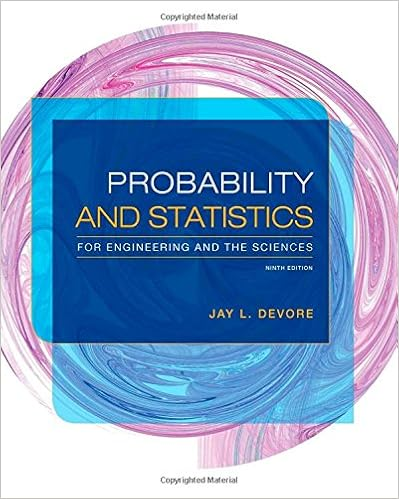# B what happens to potential energy as the car goes up

• 7
• 97% (34) 33 out of 34 people found this document helpful

This preview shows page 3 - 5 out of 7 pages.

##### We have textbook solutions for you!
The document you are viewing contains questions related to this textbook.The document you are viewing contains questions related to this textbook.
Chapter 5 / Exercise 60
Probability and Statistics for Engineering and the Sciences
DevoreExpert Verified
B.What happens to potential energy as the car goes up the hill?
3.Experiment: The kinetic energy(K) of a car describes its energy of motion. Click ResetSelect the Kvs t(kinetic energy vs. time) graph, and click PlayA.What happens to kinetic energy as the car goes down the hill? . .
B.What happens to kinetic energy as the car goes up the hill?
4.Compare: Click Reset. Set Hill 1to 80 cm, Hill 2to 60 cm, and Hill 3to 79 cm. Be sure the 50-g toy car is selected, and press Play. Sketch the Uvs t, Kvs t, and Evs tgraphs below.
##### We have textbook solutions for you!
The document you are viewing contains questions related to this textbook.The document you are viewing contains questions related to this textbook.
Chapter 5 / Exercise 60
Probability and Statistics for Engineering and the Sciences
DevoreExpert Verified
5.Draw conclusions: How are potential energy, kinetic energy, and total energy related?
6.Calculate: Gravitational potential energy (U) depends on three things: the object’s mass (m),its height (h), and gravitational acceleration (g), which is 9.81 m/s2on Earth’s surface:U= mghEnergy is measured in joules (J). One joule is equal to one 1 kg•m
2. When calculating the energy of an object, it is helpful to convert the mass and height to kilograms and meters. (Recall there are 1,000 grams in a kilogram and 100 centimeters in a meter.)A.What is the mass of the 50-gram car, in kilograms?
B.Set Hill 1to 75 cm and the other hills to 0 cm. What is the height in meters?
C.What is the potential energy of the car, in joules?
7.Calculate: Kinetic energy (K) depends on the mass and velocityof the object. (Velocity is the speed and direction of an object.) The equation for kinetic energy is:K= mvWith Hill 1set to 75 cm, click Playand allow the car to reach the bottom.A.What is the final velocity (speed) of the car, in meters per second? B.What is the kinetic energy of the car, in joules? (Use the mass in kg.) 2
•••